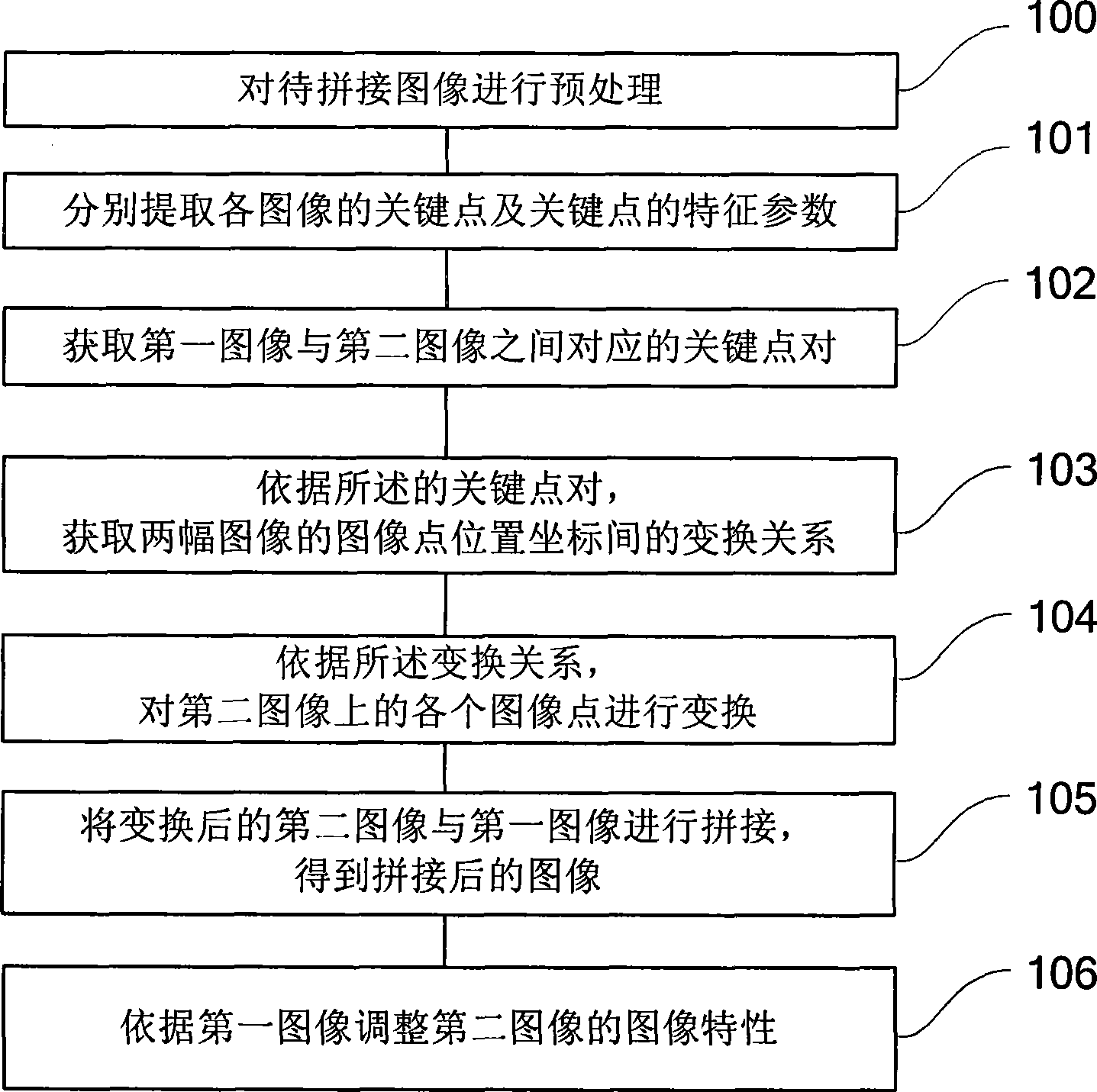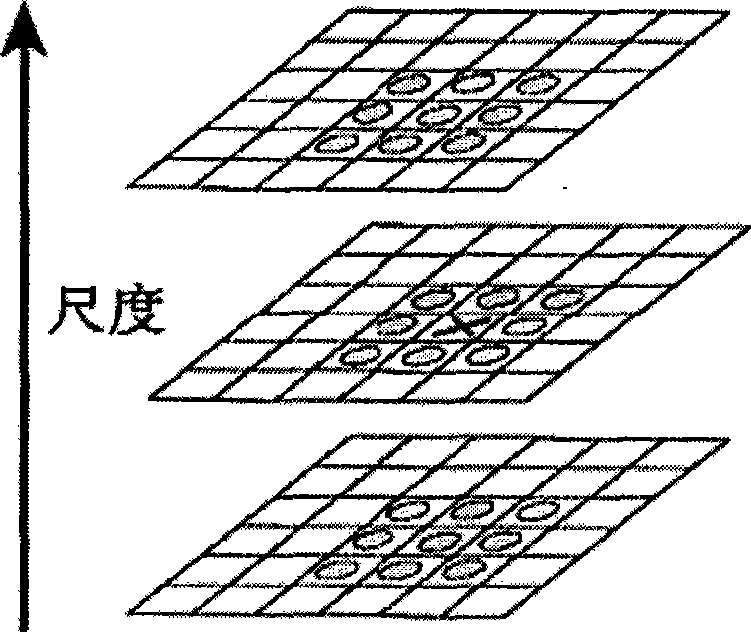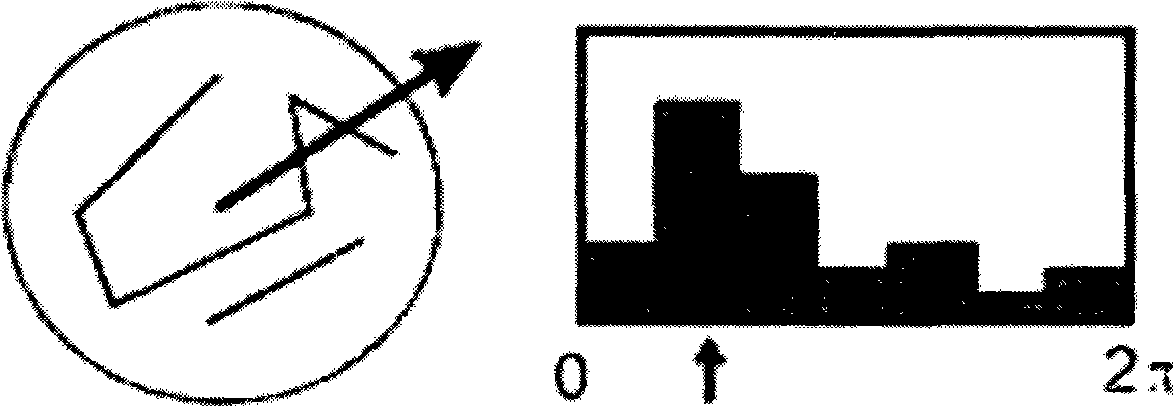# Image splicing method and apparatus

## An image stitching and image technology, applied in image data processing, graphic image conversion, instruments, etc., can solve problems such as inability to apply to most users of mobile terminals, large changes in shooting angles, and complex calculation methods.

Active Publication Date: 2009-08-12

2 Cites 40 Cited by

## AI-Extracted Technical Summary

### Problems solved by technology

 This solution does not require expensive hardware investment to obtain better panoramic images, but it requires at least 12 or more images taken from different angles to form an image sequence (more than 20% overlap between each image must be region), and it is impossible to splice images with dif...
View more

## Abstract

The invention discloses a method for mosaicking images, which comprises the following steps: extracting image key points and characteristic parameters of the key points for a first image and a second image which are to be mosaicked respectively; acquiring a corresponding key point pair between the first image and the second image; acquiring a transformation relation between the position coordinates of image points of the two images according to the key point pair; according to the transformation relation, transforming the images points in the second image; and mosaicking the transformed second image and the first image to obtain an mosaicked image. The method computes and mosaicks directly according to characteristic points of the images, is suitable for most users of mobile terminals with common photographic level and for mosaicking complex images such as rotation, zoom and angular transformation, is simple, convenient, and extremely suitable for real-time use by a mobile terminal.

Application Domain

Geometric image transformation

Technology Topic

Characteristic pointFeature parameter +2

## Image

•••## Examples

• Experimental program(4)

### Example Embodiment

 Reference figure 1 , Shows Embodiment 1 of an image stitching method of the present invention, which may specifically include:
 Step 101: For the first image and the second image to be spliced, respectively extract the key points of each image and the characteristic parameters of the key points; the first image and the second image may be selected by the user from an image library;
 Step 102: Acquire corresponding key point pairs between the first image and the second image;
 Step 103: Obtain the transformation relationship between the image point position coordinates of the two images according to the key point pair;
 For example, using polynomial fitting regression to obtain the transformation relationship:
 u = a 0 + a 1 x + a 2 y + a 3 x 2 + a 4 xy + a 5 y 2 v = b 0 + b 1 x + b 2 y + b 3 x 2 + b 4 xy + b 5 y 2
 Substituting the obtained position data (x, y) of the key point pair into the above equation, establishing a homogeneous least squares equation system and solving it can get the parameter a i , B i , Where i=0-5.
 Step 104: Transform each image point on the second image according to the transformation relationship; for example, use the above parameter a i , B i The determined transformation relation equation performs coordinate transformation on each image point on the second image;
 Step 105: Splicing the transformed second image with the first image to obtain a spliced ​​image. In fact, the transformation process in step 104 obtains the new coordinates when each image point of the second image is spliced ​​onto the first image. In the same coordinate dimension, the splicing of the two can be easily realized.
 Since the image captured by the user through the camera device (generally dynamically captured) may have problems such as blurring, noise interference, etc., in order to ensure image quality, in another preferred embodiment of the present invention, step 100 may be further included before step 101: The image to be spliced ​​is pre-processed, and the pre-processing may include noise reduction or gray-scale transformation, and if necessary, pre-processing such as coordinate transformation may also be performed.
 In addition, since the images to be spliced ​​selected by the user may have differences in the original camera angle, brightness and other acquisition conditions, the two spliced ​​images may have different image characteristics. Therefore, in another preferred aspect of the present invention, In the embodiment, after step 105, step 106 may be further included: adjusting the image characteristics of the second image according to the first image, the image characteristics including illumination characteristics or resolution, and so on. For example, adjust the light characteristics of the second image according to the light characteristics of the first image to adapt to the first image; or adjust the resolution of the second image through up-sampling or down-sampling according to the resolution of the first image to adapt to the first image. Image; or, smoothing the stitching boundary of two images, etc.
 The following briefly introduces a feasible way of acquiring image key points and their characteristic parameters: using scale-invariant feature transformation algorithm (SIFT algorithm) to extract the key points and characteristic parameters of each image.
 The result of SIFT algorithm extraction is to obtain a large number of features represented by high-dimensional descriptors distributed on different scales. A brief description of the SIFT algorithm process is as follows:
 (1) Detect extreme points in scale space
 In order to effectively detect stable key points in the scale space, the present invention can adopt the Gaussian difference scale-space (DOG scale-space), which uses different scale Gaussian difference kernels and image convolutions to construct an image pyramid to generate the required scale space .
 D(x,y,σ)=(G(x,y,kσ)-G(x,y,σ))*I(x,y)=L(x,y,kσ)-L(x,y ,Σ)
 Among them, (x, y) is the space coordinate, σ is the scale coordinate, G(x, y, σ) is the scale variable Gaussian function,
 G ( x , y , σ ) = 1 2 πσ 2 e - ( x 2 + y 2 ) / 2 σ 2
 Specifically, it is assumed that the constructed image pyramid has a total of P groups, and each group has S layers, where the images of the next group are obtained by down-sampling the images of the previous group.
 (2), precise positioning of extreme points
 In order to find the extreme points of the scale space, each sampling point must be compared with all its neighboring points to see if it is larger or smaller than the neighboring points of its image domain and scale domain. Such as figure 2 As shown, the detection point "X" in the middle is compared with its 8 neighboring points of the same scale and 9×2 points corresponding to the upper and lower neighboring scales, a total of 26 points "O" to ensure that the scale space and the two-dimensional image Extremum points are detected in all spaces.
 (3) Specify direction parameters for each key point
 In this step, the gradient direction distribution characteristics of the pixels in the neighborhood of the key point can be used to specify the direction parameter for each key point, so that the DOG operator has rotation invariance.
 m ( x , y ) = ( L ( x + 1 , y ) - L ( x - 1 , y ) ) 2 + ( L ( x , y + 1 ) - L ( x , y - 1 ) ) 2
 θ(x,y)=a tan 2((L(x,y+1)-L(x,y-1))/(L(x+1,y)-L(x-1,y)) )
 The above formula is the modulus value and direction formula of the gradient at coordinates (x, y). The scale used for L is the scale where each key point is located.
 In actual calculations, the present invention can sample in a neighborhood window centered on key points, and use a histogram to count the gradient directions of neighborhood pixels. The range of the gradient histogram is 0-360 degrees, with one bar every 10 degrees, for a total of 36 bars. The peak of the histogram represents the main direction of the neighborhood gradient at the key point, that is, the direction of the key point. image 3 It is an example of using the gradient histogram as the key point to determine the main direction when using 7 bars.
 In the histogram of gradient directions, when there is another peak that is equivalent to 80% of the energy of the main peak, this direction is regarded as the auxiliary direction of the key point. A key point may be designated to have multiple directions (one main direction, multiple auxiliary directions), which can enhance the robustness of matching.
 At this point, the key points of the image have basically been detected. Each key point includes three pieces of information: location, scale, and direction; from this, a SIFT feature area can be determined.
 (4) Generate key point descriptors
 This step is used to generate descriptors (characteristic parameters) from the above three key points of information to facilitate subsequent calculations; of course, the present invention does not need to limit the specific form of the characteristic parameters.
 First, rotate the coordinate axis to the direction of the key point to ensure rotation invariance. Next, take the 8×8 window with the key point as the center. Reference Figure 4 , The left part shows the direction of the domain gradient, the central black point is the position of the current key point, each small grid represents a pixel in the scale space where the key point neighborhood is located, the arrow direction represents the gradient direction of the pixel, and the arrow length represents the gradient Modulus value, the circle on the periphery of the figure represents the range of Gaussian weighting (the closer the pixel is to the key point, the greater the contribution of the gradient direction information).
 Then, calculate the gradient direction histogram in 8 directions on every 4×4 small block, and draw the cumulative value of each gradient direction to form a seed point, such as Figure 4 Shown in the right part (key point feature vector illustration). In this figure, a key point is composed of 2×2 4 seed points, and each seed point has 8 direction vector information. This idea of ​​combining neighborhood directional information enhances the ability of the algorithm to resist noise, and at the same time provides better fault tolerance for feature matching containing positioning errors.
 Preferably, in order to enhance the robustness of matching, the present invention can use 4×4 16 seed points to describe each key point, so that 128 data can be generated for one key point, that is, a 128-dimensional SIFT feature is finally formed. vector. At this time, the SIFT feature vector has removed the influence of geometric deformation factors such as scale change, rotation, etc., and then continue to normalize the length of the feature vector, then the influence of the illumination change can be further removed.
 It should be noted that, in addition to the foregoing implementation algorithm, the present invention may also adopt a corner detection algorithm or a matching algorithm based on edge detection. Among them, the corner detection algorithm is an image processing algorithm that directly uses the image gray level to effectively detect edges and corners.
 The following briefly introduces how to obtain the required key point pairs from the key points of the two images. For example, refer to the following table:


### Example Embodiment

 Embodiment 1
 The present invention can obtain the corresponding key point pairs between the first image and the second image in the following ways: create a Kd tree according to the key points of the first image and its characteristic parameters; for each key point of the second image, use the most The neighboring point search algorithm obtains the corresponding key points in the first image, and obtains the key point pairs.
 The KD-tree technology used in the present invention has a fast retrieval speed, and its space complexity is in a linear relationship with the dimension of the data set, and it is compatible with the implementation of the secondary memory. Therefore, it is a very effective index algorithm (which can satisfy mobile Real-time requirements of the terminal). Its basic idea is to divide the data set into two sub-data sets according to certain criteria, and then recursively divide the two sub-data sets to form a retrieval tree.
 K nearest neighbor (k-Nearest Neighbor, KNN) search algorithm is a theoretically mature method. It can be better in KD-tree to obtain one or more of the most similar (ie feature The closest sample in the space); the present invention will not be described in detail here.

### Example Embodiment

 Embodiment 2
 The present invention also obtains the corresponding key point pair between the first image and the second image in the following manner:
 (1) Create a K-d tree based on the key points and characteristic parameters of the first image;
 (2) For each key point of the second image, the nearest neighbor point search algorithm is used to obtain the nearest neighbor key point and the second neighbor key point corresponding to it in the first image;
 (3) Obtain the distance between the key point kp of the second image and the nearest key point kp1 | kp ⇔ kp 1 | , And the distance between the key point kp and the next neighbor key point kp2 | kp ⇔ kp 2 | ;
 (4) Compare the above two distances, and if the preset conditions are met, determine that the key point and the nearest key point are matched key point pairs.
 The improvement of Embodiment 2 and Embodiment 1 is that a screening and filtering step is added to exclude some feature point pairs with large matching errors. Because based on the nearest neighbor search algorithm, for a key point of the second image, at least one nearest key point can be found in the KD-tree of the first image, but it is not certain whether it is a truly better match.
 For a key point with the closest key point kp1 and the next key point kp2, the higher the matching degree with kp1, the lower the matching degree with kp2, indicating that the key point and kp1 are matched key point pairs.
 Specifically, it can be compared | kp ⇔ kp 1 | with | kp ⇔ kp 2 | , If the former is smaller and the latter is larger, it means that the better the quality of the key point kp and the nearest key point kp1 match, the lower the possibility of matching error. Therefore, the ratio of the two can be used to measure the quality of the match. | kp ⇔ kp 1 | | kp ⇔ kp 2 | λ
 It is considered that kp matches kp1, where λ is a constant and 0

## PUM## Description & Claims & Application Information

We can also present the details of the Description, Claims and Application information to help users get a comprehensive understanding of the technical details of the patent, such as background art, summary of invention, brief description of drawings, description of embodiments, and other original content. On the other hand, users can also determine the specific scope of protection of the technology through the list of claims; as well as understand the changes in the life cycle of the technology with the presentation of the patent timeline. Login to view more.
Who we serve
• R&D Engineer
• R&D Manager
• IP Professional
Why Eureka